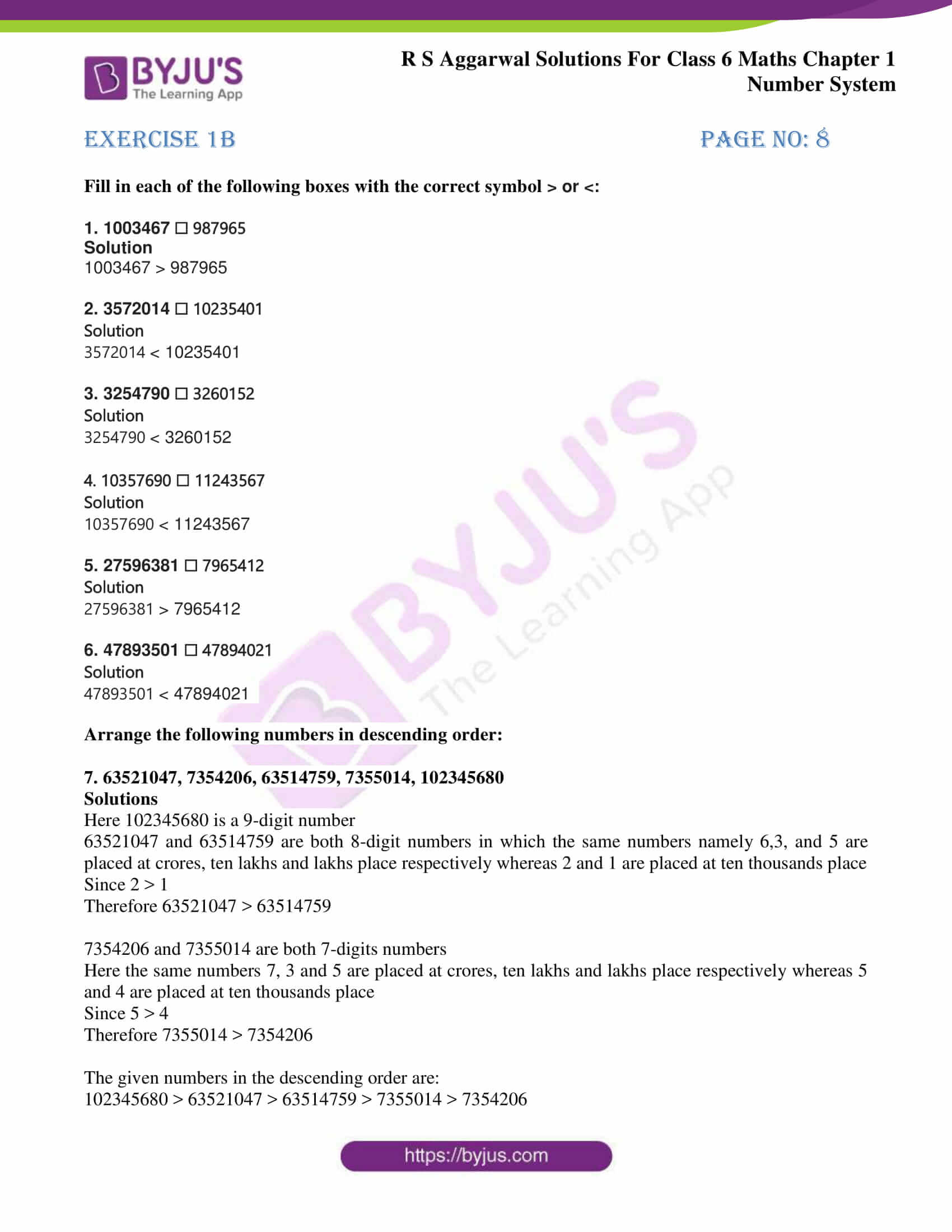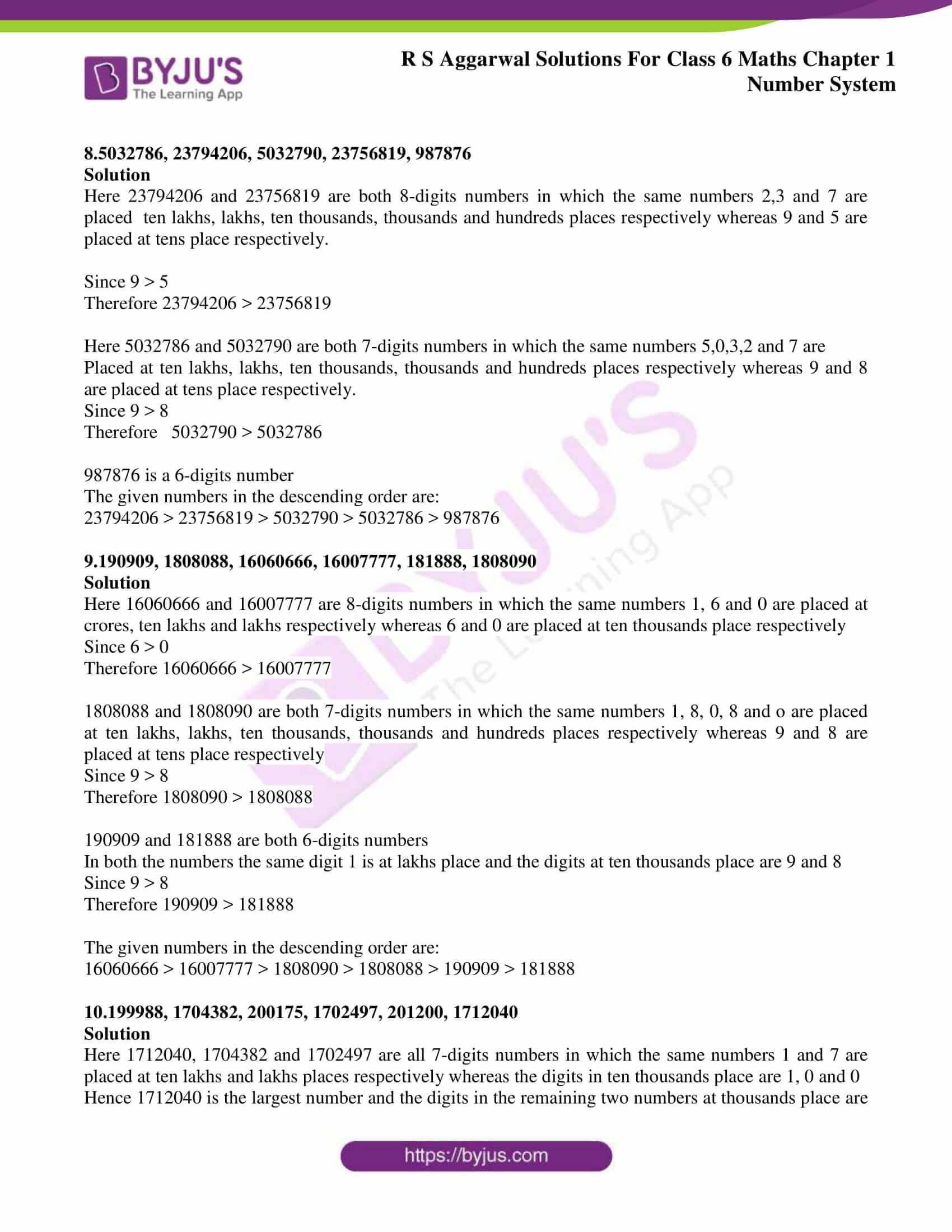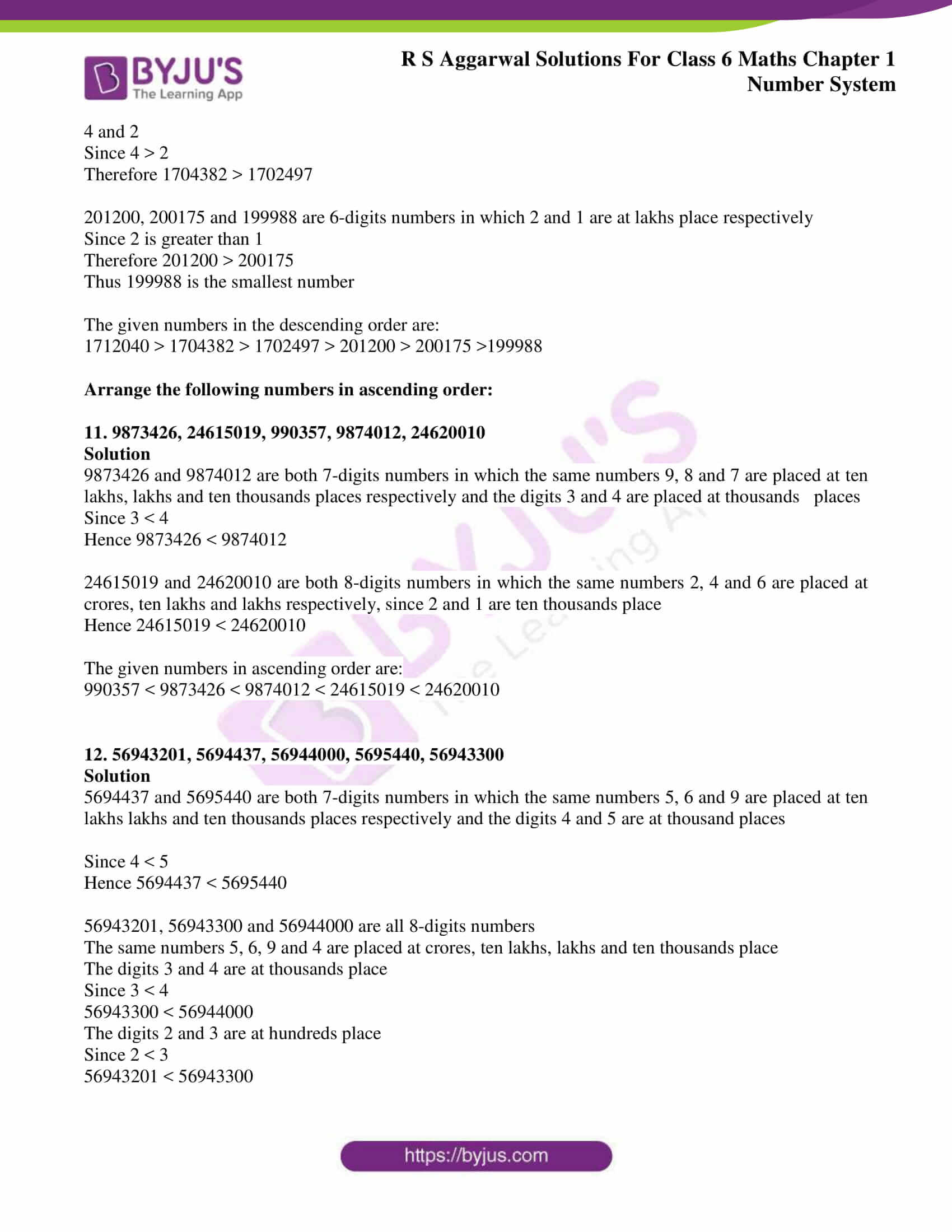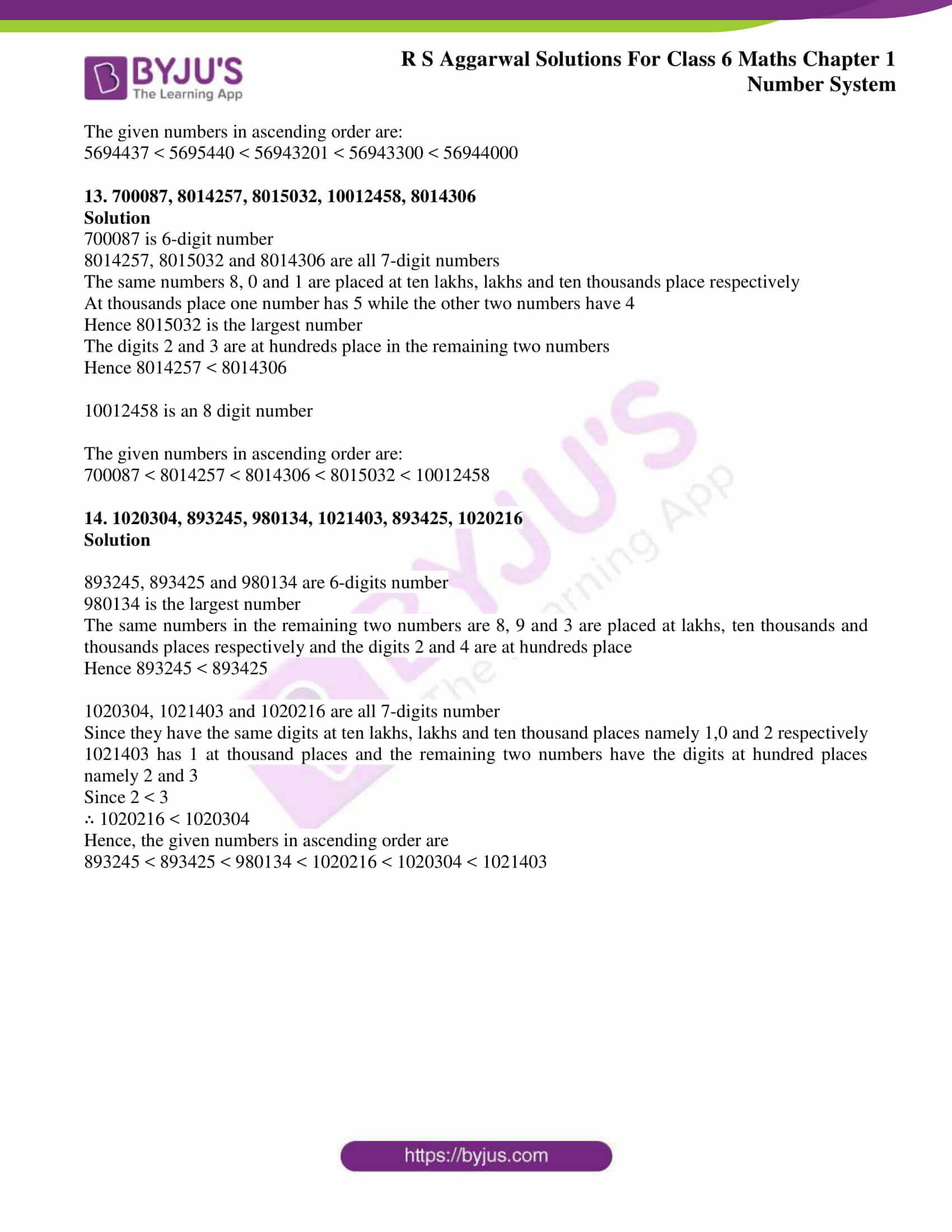# RS Aggarwal Solutions for Class 6 Chapter 1 Number System Exercise 1B

RS Aggarwal Solutions for Class 6, Chapter,1 Number System Exercise 1B PDF can be downloaded from the link which is given here. Experts at BYJU’S have solved the exercise-wise problems in depth to provide better conceptual knowledge among students. These solutions help students in solving the problems at a good speed without any difficulty. It is a vital source of study material which is important from the exam point of view. From this exercise, students learn about the concept of comparison of numbers.

Students are suggested to practice RS Aggarwal Solutions for Class 6 Chapter 1 Number System to understand the Chapter effectively without any doubts.

## Download PDF of RS Aggarwal Solutions for Class 6 Chapter 1 Number System Exercise 1B### Access answers to Maths RS Aggarwal Solutions for Class 6 Chapter 1 Number System Exercise 1B

Fill in each of the following boxes with the correct symbol > or <:

1. 1003467 ☐ 987965

Solution

1003467 > 987965

2. 3572014 ☐ 10235401

Solution

3572014 < 10235401

3. 3254790 ☐ 3260152

Solution

3254790 < 3260152

4. 10357690 ☐ 11243567

Solution

10357690 < 11243567

5. 27596381 ☐ 7965412

Solution

27596381 > 7965412

6. 47893501 ☐ 47894021

Solution

47893501< 47894021

Arrange the following numbers in descending order:

7. 63521047, 7354206, 63514759, 7355014, 102345680

Solutions

Here 102345680 is a 9-digit number

63521047 and 63514759 are both 8-digit numbers in which the same numbers namely 6,3, and 5 are placed at crores, ten lakhs and lakhs place respectively whereas 2 and 1 are placed at ten thousands place

Since 2 > 1

Therefore 63521047 > 63514759

7354206 and 7355014 are both 7-digits numbers

Here the same numbers 7, 3 and 5 are placed at crores, ten lakhs and lakhs place respectively whereas 5 and 4 are placed at ten thousands place

Since 5 > 4

Therefore 7355014 > 7354206

The given numbers in the descending order are:

102345680 > 63521047 > 63514759 > 7355014 > 7354206

8.5032786, 23794206, 5032790, 23756819, 987876

Solution

Here 23794206 and 23756819 are both 8-digits numbers in which the same numbers 2,3 and 7 are placed ten lakhs, lakhs, ten thousands, thousands and hundreds places respectively whereas 9 and 5 are placed at tens place respectively.

Since 9 > 5

Therefore 23794206 > 23756819

Here 5032786 and 5032790 are both 7-digits numbers in which the same numbers 5,0,3,2 and 7 are

Placed at ten lakhs, lakhs, ten thousands, thousands and hundreds places respectively whereas 9 and 8 are placed at tens place respectively.

Since 9 > 8

Therefore 5032790 > 5032786

987876 is a 6-digits number

The given numbers in the descending order are:

23794206 > 23756819 > 5032790 > 5032786 > 987876

9.190909, 1808088, 16060666, 16007777, 181888, 1808090

Solution

Here 16060666 and 16007777 are 8-digits numbers in which the same numbers 1, 6 and 0 are placed at crores, ten lakhs and lakhs respectively whereas 6 and 0 are placed at ten thousands place respectively

Since 6 > 0

Therefore 16060666 > 16007777

1808088 and 1808090 are both 7-digits numbers in which the same numbers 1, 8, 0, 8 and o are placed at ten lakhs, lakhs, ten thousands, thousands and hundreds places respectively whereas 9 and 8 are placed at tens place respectively

Since 9 > 8

Therefore 1808090 > 1808088

190909 and 181888 are both 6-digits numbers

In both the numbers the same digit 1 is at lakhs place and the digits at ten thousands place are 9 and 8

Since 9 > 8

Therefore 190909 > 181888

The given numbers in the descending order are:

16060666 > 16007777 > 1808090 > 1808088 > 190909 > 181888

10.199988, 1704382, 200175, 1702497, 201200, 1712040

Solution

Here 1712040, 1704382 and 1702497 are all 7-digits numbers in which the same numbers 1 and 7 are placed at ten lakhs and lakhs places respectively whereas the digits in ten thousands place are 1, 0 and 0

Hence 1712040 is the largest number and the digits in the remaining two numbers at thousands place are 4 and 2

Since 4 > 2

Therefore 1704382 > 1702497

201200, 200175 and 199988 are 6-digits numbers in which 2 and 1 are at lakhs place respectively

Since 2 is greater than 1

Therefore 201200 > 200175

Thus 199988 is the smallest number

The given numbers in the descending order are:

1712040 > 1704382 > 1702497 > 201200 > 200175 >199988

Arrange the following numbers in ascending order:

11. 9873426, 24615019, 990357, 9874012, 24620010

Solution

9873426 and 9874012 are both 7-digits numbers in which the same numbers 9, 8 and 7 are placed at ten lakhs, lakhs and ten thousands places respectively and the digits 3 and 4 are placed at thousands places

Since 3 < 4

Hence 9873426 < 9874012

24615019 and 24620010 are both 8-digits numbers in which the same numbers 2, 4 and 6 are placed at crores, ten lakhs and lakhs respectively, since 2 and 1 are ten thousands place

Hence 24615019 < 24620010

The given numbers in ascending order are:

990357 < 9873426 < 9874012 < 24615019 < 24620010

12. 56943201, 5694437, 56944000, 5695440, 56943300

Solution

5694437 and 5695440 are both 7-digits numbers in which the same numbers 5, 6 and 9 are placed at ten lakhs lakhs and ten thousands places respectively and the digits 4 and 5 are at thousand places

Since 4 < 5

Hence 5694437 < 5695440

56943201, 56943300 and 56944000 are all 8-digits numbers

The same numbers 5, 6, 9 and 4 are placed at crores, ten lakhs, lakhs and ten thousands place

The digits 3 and 4 are at thousands place

Since 3 < 4

56943300 < 56944000

The digits 2 and 3 are at hundreds place

Since 2 < 3

56943201 < 56943300

The given numbers in ascending order are:

5694437 < 5695440 < 56943201 < 56943300 < 56944000

13. 700087, 8014257, 8015032, 10012458, 8014306

Solution

700087 is 6-digit number

8014257, 8015032 and 8014306 are all 7-digit numbers

The same numbers 8, 0 and 1 are placed at ten lakhs, lakhs and ten thousands place respectively

At thousands place one number has 5 while the other two numbers have 4

Hence 8015032 is the largest number

The digits 2 and 3 are at hundreds place in the remaining two numbers

Hence 8014257 < 8014306

10012458 is an 8 digit number

The given numbers in ascending order are:

700087 < 8014257 < 8014306 < 8015032 < 10012458

14. 1020304, 893245, 980134, 1021403, 893425, 1020216

Solution

893245, 893425 and 980134 are 6-digits number

980134 is the largest number

The same numbers in the remaining two numbers are 8, 9 and 3 are placed at lakhs, ten thousands and thousands places respectively and the digits 2 and 4 are at hundreds place

Hence 893245 < 893425

1020304, 1021403 and 1020216 are all 7-digits number

Since they have the same digits at ten lakhs, lakhs and ten thousand places namely 1,0 and 2 respectively

1021403 has 1 at thousand places and the remaining two numbers have the digits at hundred places namely 2 and 3

Since 2 < 3

∴ 1020216 < 1020304

Hence, the given numbers in ascending order are

893245 < 893425 < 980134 < 1020216 < 1020304 < 1021403

## RS Aggarwal Solutions for Class 6 Maths Chapter 1 Number System Exercise 1B

Exercise 1B of RS Aggarwal Solutions for Class 6 Chapter 1 Number System gives the idea of comparison of given set of numbers. To have a better understanding of concepts, the students are advised to refer RS Aggarwal solutions. Topics which are covered in the other exercises are

• International system of numeration
• Word problems on number operations
• Estimation
• Estimating the products
• Estimating the quotients
• Roman numerals

To gain a knowledge of the above mentioned topics, the students can go through RS Aggarwal solutions which are easy to understand. Practising RS Aggarwal Solutions makes students gain expertise in Mathematics.